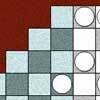Search by Topic

Resources tagged with Powers & roots similar to Rationals Between...:

Filter by: Content type:
Age range:
Challenge level:

There are 32 results

Broad Topics > Numbers and the Number System > Powers & rootsRationals Between...

Age 14 to 16 Challenge Level:

What fractions can you find between the square roots of 65 and 67?Perfectly Square

Age 14 to 16 Challenge Level:

The sums of the squares of three related numbers is also a perfect square - can you explain why?Consecutive Squares

Age 14 to 16 Challenge Level:

The squares of any 8 consecutive numbers can be arranged into two sets of four numbers with the same sum. True of false?Root to Poly

Age 14 to 16 Challenge Level:

Find the polynomial p(x) with integer coefficients such that one solution of the equation p(x)=0 is $1+\sqrt 2+\sqrt 3$.Equal Temperament

Age 14 to 16 Challenge Level:

The scale on a piano does something clever : the ratio (interval) between any adjacent points on the scale is equal. If you play any note, twelve points higher will be exactly an octave on.Archimedes and Numerical Roots

Age 14 to 16 Challenge Level:

The problem is how did Archimedes calculate the lengths of the sides of the polygons which needed him to be able to calculate square roots?Roots Near 9

Age 14 to 16 Short Challenge Level:

For how many integers 𝑛 is the difference between √𝑛 and 9 is less than 1?Number Rules - OK

Age 14 to 16 Challenge Level:

Can you convince me of each of the following: If a square number is multiplied by a square number the product is ALWAYS a square number...Negative Power

Age 14 to 16 Challenge Level:

What does this number mean ? Which order of 1, 2, 3 and 4 makes the highest value ? Which makes the lowest ?Power Countdown

Age 14 to 16 Challenge Level:

In this twist on the well-known Countdown numbers game, use your knowledge of Powers and Roots to make a target.Unusual Long Division - Square Roots Before Calculators

Age 14 to 16 Challenge Level:

However did we manage before calculators? Is there an efficient way to do a square root if you have to do the work yourself?Double Trouble

Age 14 to 16 Challenge Level:Rachel's Problem

Age 14 to 16 Challenge Level:

Is it true that $99^n$ has 2n digits and $999^n$ has 3n digits? Investigate!The Root of the Problem

Age 14 to 18 Challenge Level:

Find the sum of this series of surds.Enriching Experience

Age 14 to 16 Challenge Level:

Find the five distinct digits N, R, I, C and H in the following nomogramAge 11 to 14 Challenge Level:

Powers of numbers behave in surprising ways. Take a look at some of these and try to explain why they are true.Guesswork

Age 14 to 16 Challenge Level:

Ask a friend to choose a number between 1 and 63. By identifying which of the six cards contains the number they are thinking of it is easy to tell them what the number is.Napier's Location Arithmetic

Age 14 to 16 Challenge Level:

Have you seen this way of doing multiplication ?Fit for Photocopying

Age 14 to 16 Challenge Level:

Explore the relationships between different paper sizes.Lost in Space

Age 14 to 16 Challenge Level:

How many ways are there to count 1 - 2 - 3 in the array of triangular numbers? What happens with larger arrays? Can you predict for any size array?Pocket Money

Age 11 to 14 Challenge Level:

Which of these pocket money systems would you rather have?What an Odd Fact(or)

Age 11 to 14 Challenge Level:

Can you show that 1^99 + 2^99 + 3^99 + 4^99 + 5^99 is divisible by 5?More Magic Potting Sheds

Age 11 to 14 Challenge Level:

The number of plants in Mr McGregor's magic potting shed increases overnight. He'd like to put the same number of plants in each of his gardens, planting one garden each day. How can he do it?Deep Roots

Age 14 to 16 Challenge Level:

Find integer solutions to: $\sqrt{a+b\sqrt{x}} + \sqrt{c+d.\sqrt{x}}=1$Lastly - Well

Age 11 to 14 Challenge Level:

What are the last two digits of 2^(2^2003)?Sept 03

Age 11 to 14 Challenge Level:

What is the last digit of the number 1 / 5^903 ?Largest Number

Age 11 to 14 Challenge Level:

What is the largest number you can make using the three digits 2, 3 and 4 in any way you like, using any operations you like? You can only use each digit once.Two Many

Age 11 to 14 Challenge Level:

What is the least square number which commences with six two's?Like Powers

Age 11 to 14 Challenge Level:

Investigate $1^n + 19^n + 20^n + 51^n + 57^n + 80^n + 82^n$ and $2^n + 12^n + 31^n + 40^n + 69^n + 71^n + 85^n$ for different values of n.Diggits

Age 11 to 14 Challenge Level:

Can you find what the last two digits of the number $4^{1999}$ are?Power Crazy

Age 11 to 14 Challenge Level:

What can you say about the values of n that make $7^n + 3^n$ a multiple of 10? Are there other pairs of integers between 1 and 10 which have similar properties?Magic Potting Sheds

Age 11 to 14 Challenge Level:

Mr McGregor has a magic potting shed. Overnight, the number of plants in it doubles. He'd like to put the same number of plants in each of three gardens, planting one garden each day. Can he do it?